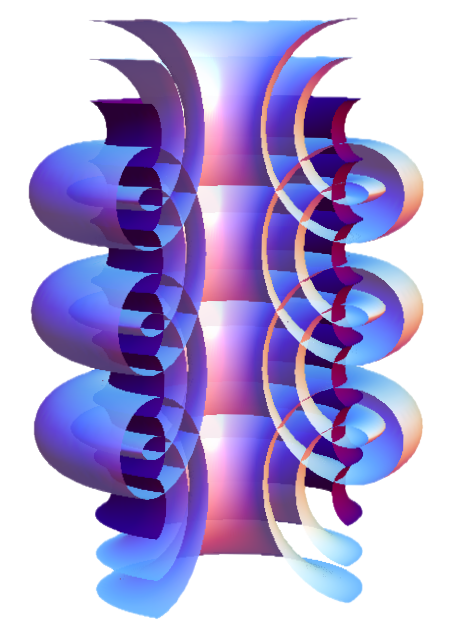# P28427-N35: Non-rigidity and Symmetry breaking

Research Project (FWF)

Funding period: 1 Oct 2016 - 31 Mar 2022 (approved 5 Oct 2015)

Field: Mathematics; Areas: Differential geometry, Integrable systemsChannel linear Weingarten surfaces
(Fig: K Mundilova)

Abstract. Properties or equations used to specify geometric objects possess certain symmetries, such as the specification of a triangle in terms of three angles, which determines a triangle up to similarity, or in terms of three edge lengths, which determines it up to Euclidean motion: these two specifications have different symmetry groups. Various theorems in geometry describe a situation, whereby a "conserved quantity", naturally associated with a geometric object, reduces the symmetry group of its defining properties: the original symmetry is broken.

Vessiot's theorem yields a classical example of symmetry breaking in differential geometry: if a surface can be deformed while preserving all properties relating to angle measurement and, at the same time, envelops a 1-parameter family of spheres, then it is piece of a cone, a cylinder or a surface of revolution. The first two properties only depend on an angle measurement, while being a cone, cylinder or surface of revolution depends on a length measurement. Thus symmetry breaking has occurred.

We will investigate relations between non-rigidity and symmetry breaking, in particular, whether deformability in more than one way invariably leads to symmetry breaking, thus generalizing Vessiot's theorem. However, our main concern will be to detect causes for symmetry breaking rather than just its occurrence, for example, by studying the appearance of the aforementioned "conserved quantities".

## People

Birgit Slama (Secretary)

Andreas Fuchs
Udo Hertrich-Jeromin
Mason Pember
Denis Polly